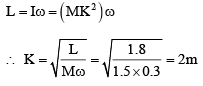Courses

# Test: Rotational Motion- 2

## 30 Questions MCQ Test Physics Class 11 | Test: Rotational Motion- 2

Description
This mock test of Test: Rotational Motion- 2 for JEE helps you for every JEE entrance exam. This contains 30 Multiple Choice Questions for JEE Test: Rotational Motion- 2 (mcq) to study with solutions a complete question bank. The solved questions answers in this Test: Rotational Motion- 2 quiz give you a good mix of easy questions and tough questions. JEE students definitely take this Test: Rotational Motion- 2 exercise for a better result in the exam. You can find other Test: Rotational Motion- 2 extra questions, long questions & short questions for JEE on EduRev as well by searching above.
QUESTION: 1

### Four masses are fixed on a massless rod as shown fig. The moment of inertia about the axis P is about :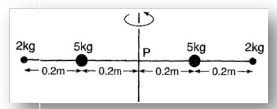Solution:

Moment of inertia=m X r2
Here origin is chosen at the point through which the axis passes the rod. Using the formula total moment of inertia about axis is,
=2 X 5 X (0.2)2 + 2 X 2 X (0.4)2
=1.04kgm2

QUESTION: 2

### An inclined plane makes an angle of 30o with the horizontal. A ring rolling down this inclinedplane from rest without slipping has a linear acceleration equal to :

Solution:

We know for a rolling body acceleration down an inclined plane, a = g.sinθ / (1 + I/mR2)
Where I is body's moment of inertia. Here, I = mR2
Thus just by putting the values to the formula we get
a = g.sin 30° / 2
= g/4

QUESTION: 3

### A disc of radius b and mass m rolls down an inclined plane of vertical height h. the translational speed when it reaches the bottom of the plane will be

Solution:

The difference in the potential of the body when it rolls down through a vertical height h, is mgh.
As the KE at the top point is zero and let say KE at bottom is ½ mv2 + ½ Iw2
Where m is its mass, I is its moment of inertia, I = ½ mr2
Where r is its radius, v is its gained translational speed and w is its gained angular speed.
w = v/r
Hence equating PE and KE gives
mgh = ½ mv2 + ½ Iw2
That is mgh = ½ mv2 + ½ mr2.(v/r)2
We get mgh = ½  mv2 + ¼  mv
Thus we get v = √gh/3

QUESTION: 4

The kinetic energy T of a particle of mass m moving in a circle of radius r varies with the distance traced, S as T = KS2. The tangential acceleration of the particle is

Solution: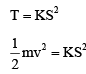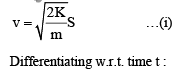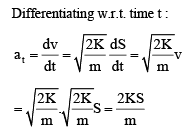QUESTION: 5

Two rings of radius R and nR made up of same material have the ratio of moment of inertia about an axis passing through centre is 1 : 8. The value of n is

Solution:
Circular loops (rings) of the wire:
Radii:     r₁ =  r    ,   r₂ = n * r

Mass of the wire per unit length = d
Mass of loop₁ = m₁ = 2 π r₁ * d = 2π r d
Mass of loop₂ = m₂ = 2 π r₂ * d  = 2π n r d

Moment of Inertia of a circular loop about an axis perpendicular to the plane of the loop and passing through the center of the loop :
I = M R^2,
M = Mass,     R = radius

MOI of given loops:
I₁ = (2π r d) r^2 = 2 π d r^3
I₂ = (2π n r d) (n r)^2 = 2 π d n^3 r^3

Given   I₂ = 8 I₁
=>  2π d n^3 r^3 = 8 * 2 π d r^3
=>  n^3 = 8
=>  n = 2.

QUESTION: 6

Four point masses, each of value m, are placed at the corners of a square ABCD of side l. The moment of inertia of this system about an axis passing through A and parallel to BD is

Solution: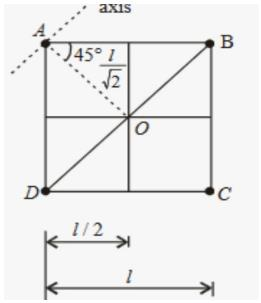IA​=0 (on the Axis .)
IB​=ID​=mr2
=m(​l​/√2)2
=ml2/​2
IC​=m(√2​l)2
IC​=2ml2
I=IA​+IB​+IC​+ID
I=0+ml2/2​+ml2/2​+2ml2
I=3ml2

QUESTION: 7

A solid sphere of radius R has moment of inertia I about its diameter. It is melted into a disc of radius r and thickness t. If its moment of inertia about the tangential axis (which is perpendicular to plane of the disc), is also equal to I, then the value of r and t are (respectively)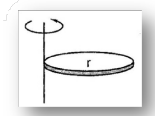Solution:

We must apply the parallel axis theorem to find moment of inertia of the disc about the given axis. On equating the moment of inertia of sphere and disc we will get a relation between "r" and "R". Also, mass remains constant and from here we can get the relation between "t" and "R" (for disc volume = area x thickness).
Isphere=Idisc+mr2
⇒ 2/5mR2=1/2mr2+mr2
⇒ 2mR2/5=3mr2/2
⇒ r=2R/√15
On conserving mass:
⇒ (4/3πR3)ρ=(πr2t) ρ
⇒ 4/3πR3=π(4R2/15)t
⇒ t=5R

QUESTION: 8

Point C is the centre of the rigid body shown in fig. Find the total torque acting on the body

about point C.(CW - clockwise ACW - anticlockwise)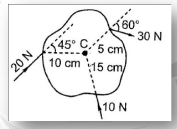Solution: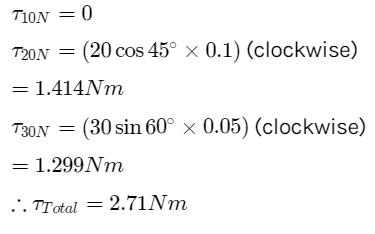QUESTION: 9

A thin rod of length 4 l , mass 4 m is bent at the points as shown in the fig. What is the moment of inertia of the rod about the axis passing through point O and perpendicular to the plane of the paper :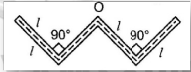Solution:

Since the mass of rod is 54m and length is 4l.
so mass C = m
and length of AB = BO=OC= CD = l
WE, know moment of Inertia of a rod about to end = ml2​/3
So, moment of Inertia of AB, BO, OC, CD about B, O, O, C respectively = ml2/3​
From parallel axis theorem
Moment of Inertia of AB about O.
=ml2/3​+ml2=4ml2​/3
Similarly od CD about O   =4ml2/3​
SO moment of Inertia Rod about O
=ml2/3​+ml2/3​+4ml2/3​+4ml2/3​
=10ml2/3​

QUESTION: 10

In the pulley system shown, if radii of the bigger and smaller pulley are 2 m and 1 m respectively and the acceleration of block ‘A’ is 5 m/s2 in the downward direction , then the acceleration of block B will be :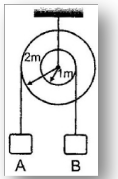Solution:

Since both pulley are connected to each other. So, their angular acceleration are equal.
⇒α1​=α2⇒​a1/ r1=a2/ r2 because α=a/r
⇒a1/1​​=5/2(Given, a2=5m/s2)
⇒a1=5/2 ​m/s2
Therefore, D is the correct option.

QUESTION: 11

An equilateral prism of mass m rests on a rough horizontal surface with coefficient of friction m . A horizontal force F is applied on the prism as shown in the fig. If the coefficient of friction is sufficiently high so that the prism does not slide before toppling, then the minimum force required to topple the prism is :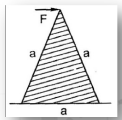Solution:

As the force is applied towards right the prism will topple at O. Now, for the prism to topple the torque due to force "F" must be greater than or equal to the torque due to weight "Mg" about the point O.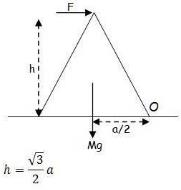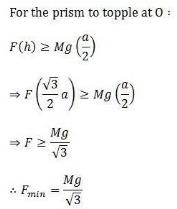QUESTION: 12

A uniform rod of mass m and length L lies radially on a disc rotating with angular speed ω in a horizontal plane about its axis. The rod does not slip on the disc and the centre of the rod is at a distance R from the centre of the disc,. Then the kinetic energy of the rod is

Solution:

By theorem of parallel axes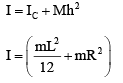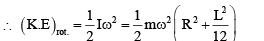QUESTION: 13

A uniform rod of length L and mass M is bent to make a reactangle PQRS such that QR/PQ = 1/2 Moment of inertia of the rectangle about the side QR can be expressed as :

Solution:

Firstly, using the relations given in the questions, we will find the length and mass of the partsQUESTION: 14

A uniform disc of radius 20 cm and mass 2 kg is fixed at its centre and can rotate about an axis through the centre and perpendicular to its plane. A massless cord is round along the rim of the disc. If a uniform force 2 newton is applied on the cord, tangential acceleration of a point on the rim of the disc will be

Solution: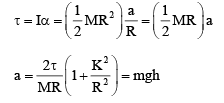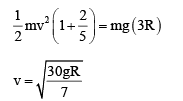QUESTION: 15

Fig shows a hemisphere of radius 4R A ball of radius R is released from position P. It rolls . A ball of without slipping along the inner surface of the hemisphere. Linear speed of its centre of mass when the ball is at position Q is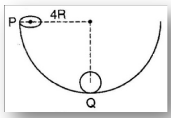Solution:
QUESTION: 16

Three point masses, each m, are placed at the vertices of an equilateral triangle of side ‘α" Moment of inertia of the system about the axis COD which passes through the mass at O and lies in the plane of triangle and perpendicular to OA is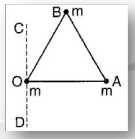Solution:

The two masses are at a distance of “a” and “a/2” so,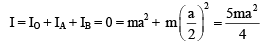QUESTION: 17

An object of mass m is projected with a velocity u at an angle 45° with the horizontal. When  the object is at maximum height, its angular momentum about the point of projection is

Solution:
max height ,H= u^2sin^2θ/2g

velocity at max height = ux= ucosθ

angular momentum= muxH

and putting θ =45degree

angular momentum = mu^3/g4√2
QUESTION: 18

A spherical ball of mass 2 kg is rolling without slipping with a speed 4 m/s on a rough floor. Its rotational kinetic energy is :

Solution: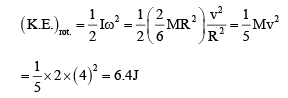QUESTION: 19

Fig shows a flywheel of radius 10 cm. Its moment of inertia about the rotation axis is 0.4 kg m2. A massless string passes over the flywheel and a mass 2 kg is attached at its lower end. Angular acceleration of the mass in rad/s2  is nearly.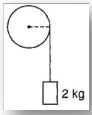Solution: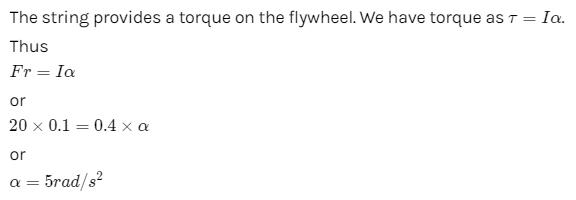I took g = 10 but if g = 9.8 is taken then we get the exact answer.

QUESTION: 20

Fig. shows a uniform rod of length  l and mass M which is pivoted at end A such that it can rotate in a vertical plane. The free end of the rod B is initially vertically above the pivot and then released. As the rod rotates about A, its angular acceleration when it is inclined to horizontal at angel q is :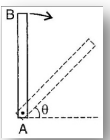Solution:
QUESTION: 21

A thin wire of length L and uniform linear mass density r is bent into a circular loop with centre O as shown. The moment of inertia of the loop about the axis XX is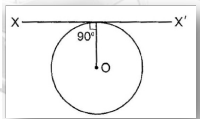Solution:
QUESTION: 22

A metal wire of mass m and length l is rotated in a circle by holding it at one end. The breaking stress of wire is F and its area of cross-section is a. calculate the maximum frequency of rotation at which it breaks

Solution:
QUESTION: 23

A cylinder of mass m radius R is spinned in a clockwise direction with angular velocity ωo and then gently placed on an inclined plane for which coefficient of friction μ = tanθ, θ is the angle of inclined plane with the horizontal. The cylinder continues to spin without failing for time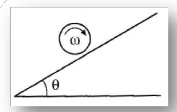Solution:
QUESTION: 24

A solid sphere of mass M, radius R and having moment of inertia about an axis passing  through the centre of mass as I, is recast into a disc of thickness t, whose moment of inertia about an axis passing through its edge and perpendicular to its plane remains I. then, radius of the disc will be :

Solution: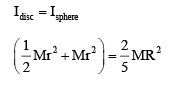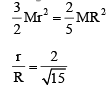QUESTION: 25

A cylinder is placed on a rough inclined surface of inclination q. Minimum value of coefficient of static friction between the cylinder and the surface so that the cylinder rolls without slipping is :

Solution: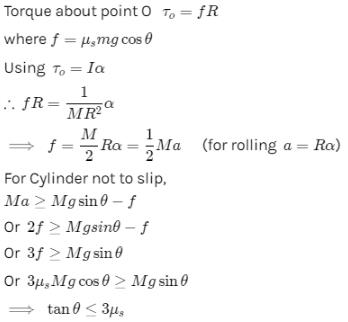QUESTION: 26

A tube of length L is filled completely with an incompressible liquid of mass M and is closed at both ends. The tube is then rotated in a horizontal plane about one of its ends with a uniform angular velocity w. Force exerted by  the liquid at the other end is :

Solution:

The force exerted by liquid at the other end is the centripetal force due to rotation. The case can be treated as rotation of the center of mass of the liquid at distance L/2 from the axis of rotation.
Hence force exerted by liquid at the end =QUESTION: 27

A small object of uniform density rolls up a curved surface with an initial velocity v. it reaches up to a maximum height of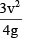with respect to the initial position. The object is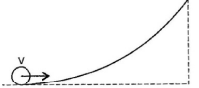Solution: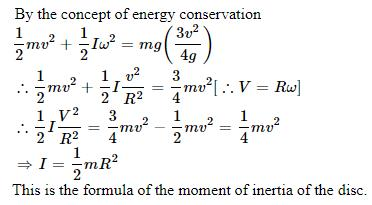QUESTION: 28

A solid sphere of mass 2 kg rolls on a smooth horizontal surface at 10 m/s. it then rolls up a smooth inclined plane of inclination 30° with the horizontal. The height attained by the sphere before it stops is

Solution:

Using the energy conservation
we know ωr = v = 10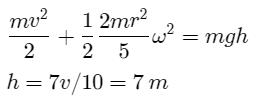QUESTION: 29

A solid sphere is rolling without slipping on a horizontal surface. The ratio of its rotational kinetic energy to its translational kinetic energy is

Solution: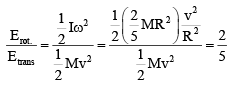QUESTION: 30

A body of mass 1.5 kg rotating about an axis with angular velocity of 0.3 rad s-1 has an angular momentum of 1.8 kg m2s-1. The radius of gyration of the body about an axis is :

Solution:

Angular momentum is given by